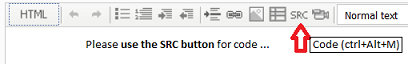# Sequential precedence of indicators in an EA ?

Hi to you all in the forum,

I am now experimenting and studying mql5 for several months and despite a lot of research I could not find an example for my problem.

The trading idea is that a buy signal occurs if one of two moving average signals are positive and the RSI has first fallen below 30, then risen above 60 and then fallen to below 45 again before it rises to 50 or above.

The problem is to determine that the RSI conditions have to be fulfilled one after the other in a sequential precedence. Has anyone of you an idea how to solve this problem? I have copied my EA for you so that you can see my coding. Thanks a lot !

Andre

//+------------------------------------------------------------------+

//|                                                       André .mq5 |

//|                                             https://www.mql5.com |

//+------------------------------------------------------------------+

string signal;

string EURUSD;

int zaehler;

extern int MagicNumber = 55557;

int ticket;

//+------------------------------------------------------------------+

//| Expert initialization function                                   |

//+------------------------------------------------------------------+

int OnInit()

{

//---

//---

return(INIT_SUCCEEDED);

}

//+------------------------------------------------------------------+

//| Expert deinitialization function                                 |

//+------------------------------------------------------------------+

void OnDeinit(const int reason)

{

//---

}

//+------------------------------------------------------------------+

//| Expert tick function                                             |

//+------------------------------------------------------------------+

void OnTick()

{

//---

}

//+------------------------------------------------------------------+

//---

//| expert start function                                            |

//+------------------------------------------------------------------+

int start()

{

//----

//+------------------------------------------------------------------+

//+------------------------------------------------------------------+

double ma = iMA(EURUSD,0,135,0,MODE_SMA,PRICE_CLOSE,1);

double mat1 = (iMA(EURUSD,0,135,0,MODE_SMA,PRICE_CLOSE,0))/(iMA(NULL,0,135,1,MODE_SMA,PRICE_CLOSE,1));

double mat30 = (iMA(EURUSD,0,135,30,MODE_SMA,PRICE_CLOSE,0))/(iMA(NULL,0,135,31,MODE_SMA,PRICE_CLOSE,1));

double r30 = iRSI(EURUSD,30,18,PRICE_CLOSE,0);

double r60 = iRSI(EURUSD,0,18,PRICE_CLOSE,0);

double r45 = iRSI(EURUSD,0,18,PRICE_CLOSE,0);

double r50 = iRSI(EURUSD,0,18,PRICE_CLOSE,0);

int r30min = iLowest(r30,0,3,100,25);

int r60max = iHighest(r60,0,3,80,10);

int r40min = iLowest(r45,0,3,20,1);

if(mat1 > 0 || mat30 > 0 && r30min <= 30 && r60max >= 60 && r40min <= 45 && r50 > 50)

{

signal = "long";

}

else   {

}

return(0);

}

Naseskippy: The problem is to determine that the RSI conditions have to be fulfilled one after the other in a sequential precedence. Has anyone of you an idea how to solve this problem?
1.Play video Please edit your post. For large amounts of code, attach it.

2. ```int r30min = iLowest(r30,0,3,100,25);
int r60max = iHighest(r60,0,3,80,10);
int r40min = iLowest(r45,0,3,20,1);```
This is utter babel. RTFM.
3. RSI has first fallen below 30, then risen above 60 and then fallen to below 45 again before it rises to 50 or above.
You haven't stated a concrete statement. What happens if it goes above 60 and then again below 30. Do you reset or not?
4. Do it exactly as you state.
```enum rsiState {IDLE, BELOW30, ABOVE60, BELOW45, ABOVE50;
static rsiState state=IDLE;
if(     r30 < 30 &&(state == IDLE ||
state == ABOVE50)) state = BELOW30;
else if(r30 > 60 && state == BELOW30)  state = ABOVE60;
else if(r30 < 45 && state == ABOVE60)  state = BELOW45;
else if(r30 > 50 && state == BELOW45)  state = ABOVE50;```

Naseskippy:

Hi to you all in the forum,

I am now experimenting and studying mql5 for several months and despite a lot of research I could not find an example for my problem.

The trading idea is that a buy signal occurs if one of two moving average signals are positive and the RSI has first fallen below 30, then risen above 60 and then fallen to below 45 again before it rises to 50 or above.

The problem is to determine that the RSI conditions have to be fulfilled one after the other in a sequential precedence. Has anyone of you an idea how to solve this problem? I have copied my EA for you so that you can see my coding. Thanks a lot !

NB! Very Important Note! This is a MQL4 forum, not MQL5! If your code is truly MQL5, you should place your queries at the MQL5 Forum!

Although in your statement you refer to MQL5 and in the EA code you use the ".mq5" extension, you are using MQL4 code in it. You cannot mix exclusive MQL5 and MQL4 concepts - only if they are common to both. You must decide which one you wish to use - either your code is ".mq4" (used in MetaTrader 4) or ".mq5" (used in MetaTrader 5).

For example, iLowest() and iHighest() is only available in MQL4, not in MQL5.

Only after you decide which one to use (MQL4 or MQL5) can we address the many other errors you have in your code!